3.2 Generating extraterrestrial terrain

Techniques such as Fourier filtering [1, 9], random midpoint displacement , Poisson faulting  often creates more or less realistic fractal forgeries of nature. They do not consider over that the real terrain is not self-similar or identical from top to bottom. In thus generated terrains we do not find craters, streaks, and other features typical for "extraterrestrial terrain".

Pickover  uses a logistic function (2) for simulating these features.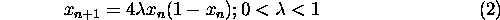This function was originally introduced by Belgian sociologist and mathematician P.F. Verhulst to model the growth of population limited by finite resources. If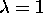, the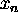are "random" numbers from the interval (0,1) with U-shaped probability distribution (see Figure 7 a) ). Using transformation of the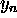according to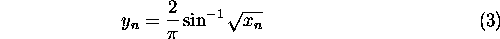we can thoughtas random number generator with the numbers uniformly distributed over the interval (0,1) (see Figure 7 a)). Pickover  recommended another transformation given by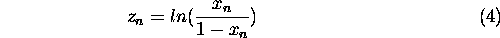Figure 7: a) Distribution ofandb) Distribution ofThis transformation may operate on the logistic variable and Collins et al.  shows that the transformation (4) generates a sequence of "random" numbers with a near-Gaussian distribution (see Figure 7 b) ). In the next step we extend equations (3) and (4) to 3 dimensions. We assumedistribution to be a radius anddenotes an angle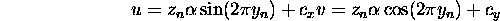Variables u and v in equation (5) are thus coordinates ofandin polar coordinate system. In every iteration the u and v coordinates are evaluated and an element P(u,v) in 2D array is incremented by one. After many iterations array P(u,v) viewed as 3D surface resembles a circular ridge with center at. Each ridge has a near Gaussian cross-section. The diameter of the crater is controlled by variable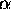i.e. the distance from center to the ridge.

Advantage of this method is that it can be used for tuning of already created terrains. The near-Gaussian numbers are easy to incorporate into software for stochastic modeling and produce structures that look like a crater with self-similar details.

Ivo Marak - marak@sgi.felk.cvut.cz